Next: Heat and work Up: Statistical mechanics Previous: Probability calculations

## Behaviour of the density of states

Consider an isolated system in equilibrium whose volume is, and whose energy lies in the rangeto. Let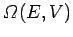be the total number of microscopic states which satisfy these constraints. It would be useful if we could estimate how this number typically varies with the macroscopic parameters of the system. The easiest way to do this is to consider a specific example. For instance, an ideal gas made up of spinless monatomic particles. This is a particularly simple example, because for such a gas the particles possess translational but no internal (e.g., vibrational, rotational, or spin) degrees of freedom. By definition, interatomic forces are negligible in an ideal gas. In other words, the individual particles move in an approximately uniform potential. It follows that the energy of the gas is just the total translational kinetic energy of its constituent particles. Thus,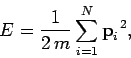(104)

whereis the particle mass,the total number of particles, and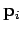the vector momentum of the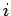th particle.

Consider the system in the limit in which the energyof the gas is much greater than the ground-state energy, so that all of the quantum numbers are large. The classical version of statistical mechanics, in which we divide up phase-space into cells of equal volume, is valid in this limit. The number of stateslying between the energiesandis simply equal to the number of cells in phase-space contained between these energies. In other words,is proportional to the volume of phase-space between these two energies: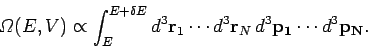(105)

Here, the integrand is the element of volume of phase-space, with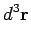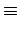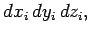(106)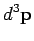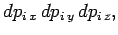(107)

where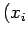,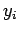,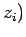and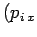,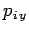,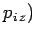are the Cartesian coordinates and momentum components of theth particle, respectively. The integration is over all coordinates and momenta such that the total energy of the system lies betweenand.

For an ideal gas, the total energydoes not depend on the positions of the particles [see Eq. (104)]. This means that the integration over the position vectorscan be performed immediately. Since each integral over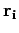extends over the volume of the container (the particles are, of course, not allowed to stray outside the container),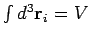. There aresuch integrals, so Eq. (105) reduces to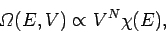(108)

where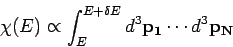(109)

is a momentum space integral which is independent of the volume.

The energy of the system can be written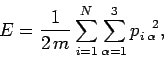(110)

since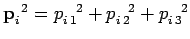, denoting the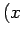,,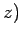components by (1, 2, 3), respectively. The above sum contains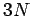square terms. For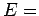constant, Eq. (110) describes the locus of a sphere of radius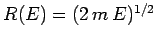in the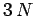-dimensional space of the momentum components. Hence,is proportional to the volume of momentum phase-space contained in the spherical shell lying between the sphere of radius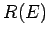and that of slightly larger radius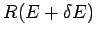. This volume is proportional to the area of the inner sphere multiplied by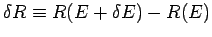. Since the area varies like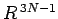, and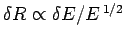, we have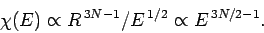(111)

Combining this result with (108) yields(112)

whereis a constant independent ofor, and we have also made use of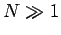. Note that, since the number of degrees of freedom of the system is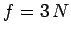, the above relation can be very approximately written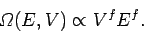(113)

In other words, the density of states varies like the extensive macroscopic parameters of the system raised to the power of the number of degrees of freedom. An extensive parameter is one which scales with the size of the system (e.g., the volume). Since thermodynamic systems generally possess a very large number of degrees of freedom, this result implies that the density of states is an exceptionally rapidly increasing function of the energy and volume. This result, which turns out to be quite general, is very useful in statistical thermodynamics.Next: Heat and work Up: Statistical mechanics Previous: Probability calculations
Richard Fitzpatrick 2006-02-02